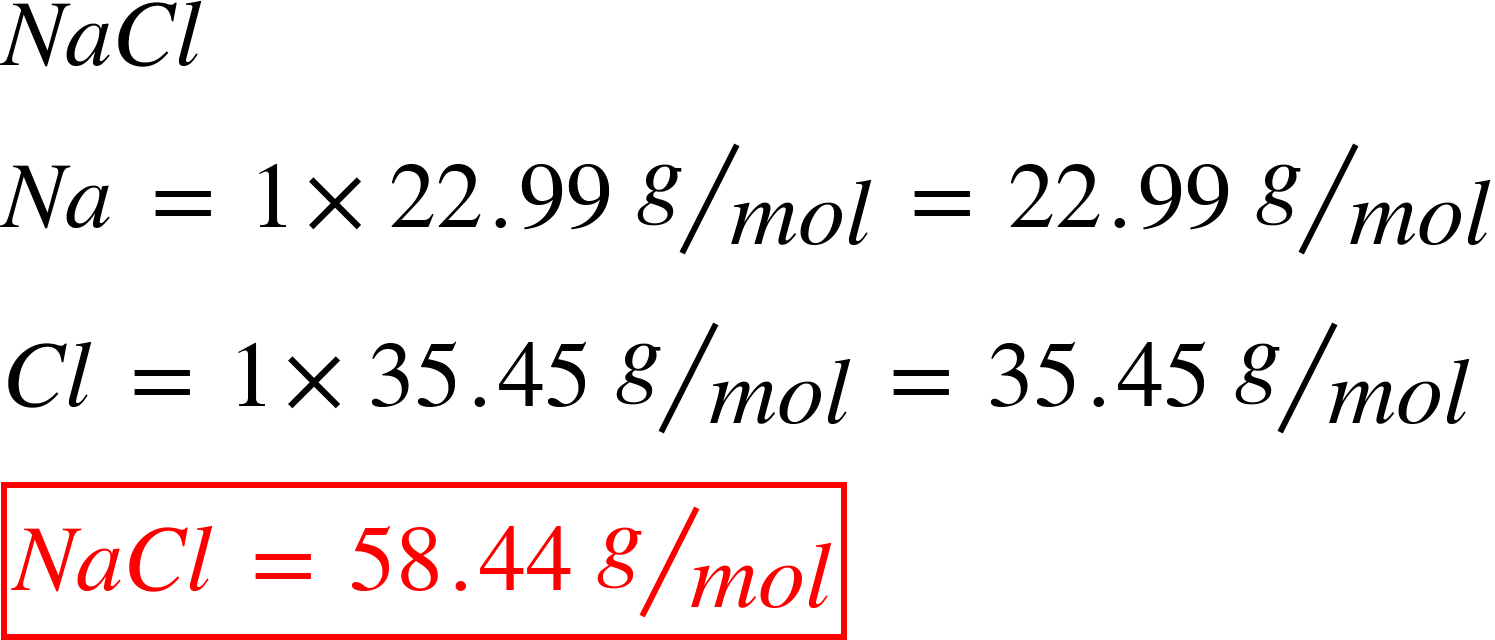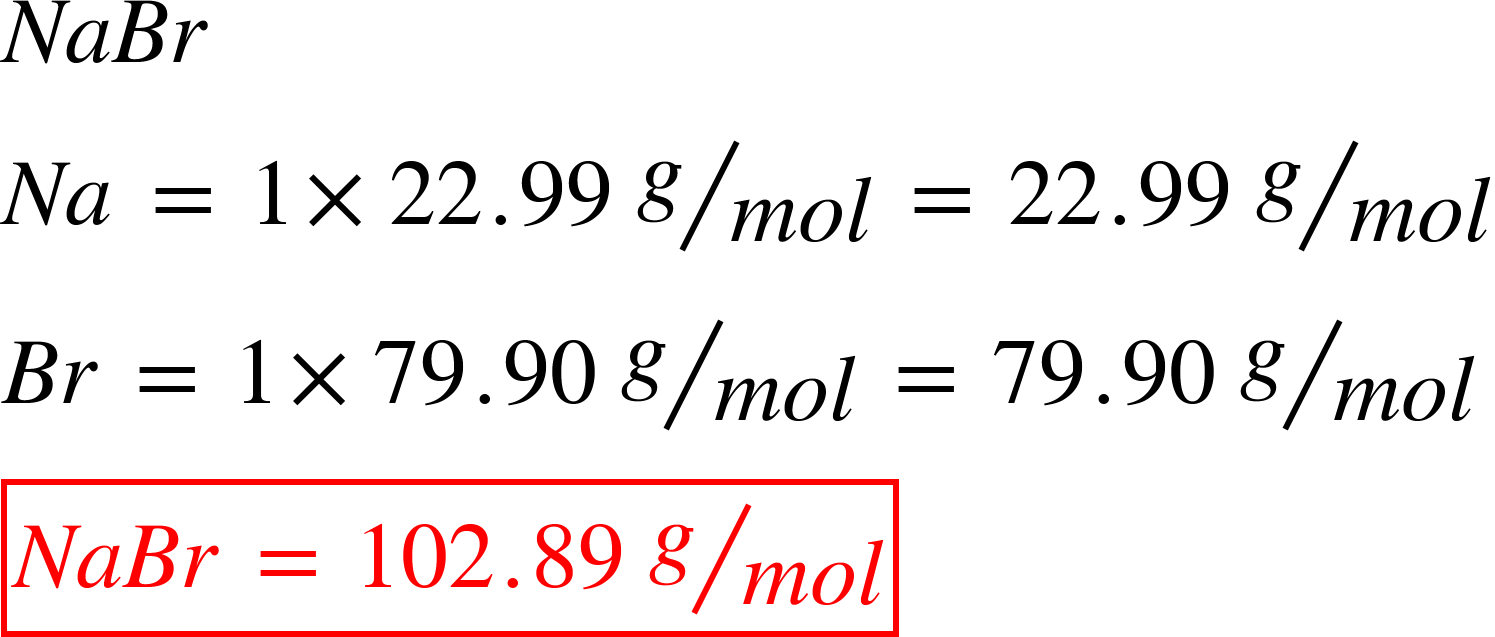# Problem: A mixture of NaCl and NaBr has a mass of 2.05 g and is found to contain 0.75 g of Na.What is the mass of NaBr in the mixture?

###### FREE Expert Solution

This is a problem where, we have two unknowns.

The mass of Na that is found in the sample comes from both the mass of NaCl and mass of NaBr.

To solve this problem, we will need molar masses of NaCl and NaBr.

Molar mass of NaClMolar mass of NaBr80% (500 ratings)###### Problem Details

A mixture of NaCl and NaBr has a mass of 2.05 g and is found to contain 0.75 g of Na.

What is the mass of NaBr in the mixture?